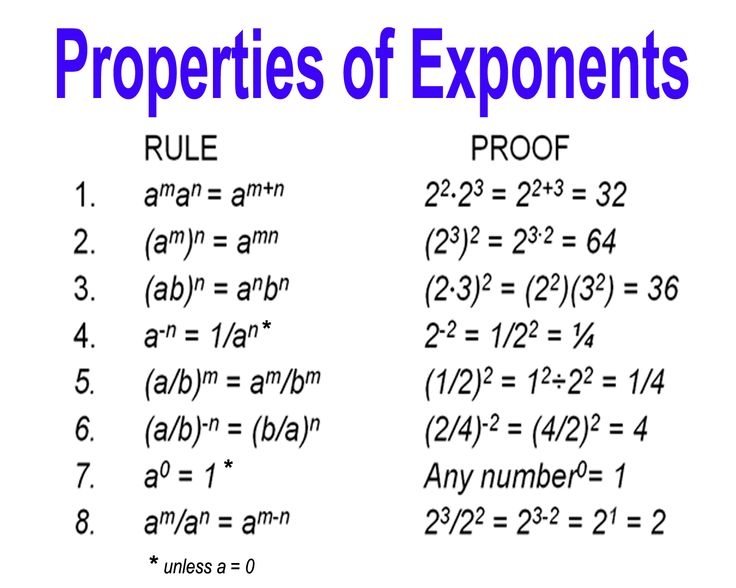## Pre-Algebra Tutorial

#### Intro

Exponents that are whole numbers are straight forward: 5 to the second is 25 and 6 to the third is 216. 5 to the second is also known as 5 squared and 6 to the third is also known as 6 cubed. Why? Because 5×5= 25= 5 squared and 6x6x6= 216= 6 cubed. This is the most basic form of exponents. Now we throw together numbers and equations:Don’t be scared; these are the exponent properties and a very helpful guide in solving and simplifying equations. Let us take the first rule and apply it: 5 to the second times 5 to the sixth would be 5 to the eighth. Rule 2: 4 to the third to the fifth is 4 to the fifteenth. Rule 3: (4×6)to the second is 4 to the second multiplied by 6 to the second. Rule 4: 5 to the negative third is one divided by 5 to the third. Rule 5: (4/7) to the third is 4 to the third divided by 7 to the third. Rule 6: (4/9) to the negative second is (9/4) to the second. Rule 7: any number (except zero) to the power of zero will equal one. Rule 8: 7 to the sixth divided by 7 to the second is 7 to the fourth.

That’s a lot. Sometimes there will be a rule within a rule. For example: 3 to the second divided by 3 to the negative third is what? Well there seems to be rules 4 and 8 in this equation. DON’T OVER THINK IT! Just use rule 8: 2-(-3)=5. So this equation is actually equal to 3 to the fifth.

TIP: When using a calculator, be very cautious as to where the parenthesis, negative, and exponent buttons should be used. It will make a huge difference for some of these rules.

Applying a function for a number is usually how equations will be written: x to third or y to the negative fifth. Do not let this confuse you. They are simply letters in place of numbers that do not yet have value. In the future, more tools will be available for you to solve these functions. For now, this will help better understand the simplification of these functions.

#### Sample Problem

Do not calculate the final number; find the simplified version.
1. 3 to the third multiplied by 3 to the fifth

2. 2 to the negative second divided by 2 to the fifth

3. 4 to the third to the fourth

4. 12 to the zero power

5. (4×7) to the sixth

6. (6/2) to the negative fourth

7. 10 to the negative third

8. (9/5) to the third

9. 9 to the negative third divided by 9 to the power of zero

#### Solution

1. 3 to the eighth.
Rule 1: add the exponents 3+5

2. 2 to the negative seventh.
Rule 8: subtract the exponents -2-5

3. 4 to the twelfth.
Rule 2: multiply the exponents 3×4

4. 1
Rule 7: any number (except zero) to the power of zero is 1

5. 4 to the sixth multiplied by 7 to the sixth.
Rule 3: take the outside exponent of 6 and apply it to each number inside the parenthesis

6. (2/6) to the fourth.
Rule 6: Switch the numbers inside the parenthesis to make the exponent positive

7. 1 divided by 10 to the third.
Rule 4: take one and divide it by the original number to make the exponent positive

8. 9 to the third divided by 5 to the third.
Rule 5: Take the outside exponent and apply it to each number

9. 1 divided by 9 to the third
Rule 7: the 9 to the power of zero becomes one
Rule 4: take one and divide it by the original number to make the exponent positive

# About The Author

 Zachary P Math, Financing, And Accounting I am a recent graduate from Kent State University with an Associate of Applied Business and a Bachelor of Business Administration. During my studies, I excelled at math, financing, and accounting and was offered tutoring jobs at the university. Unfortunately, I had to decline the jobs at the time du...
6 SubjectsKnowRo Tutor
8 Tutorials
Ashtabula, OH
Get Tutoring Info

^